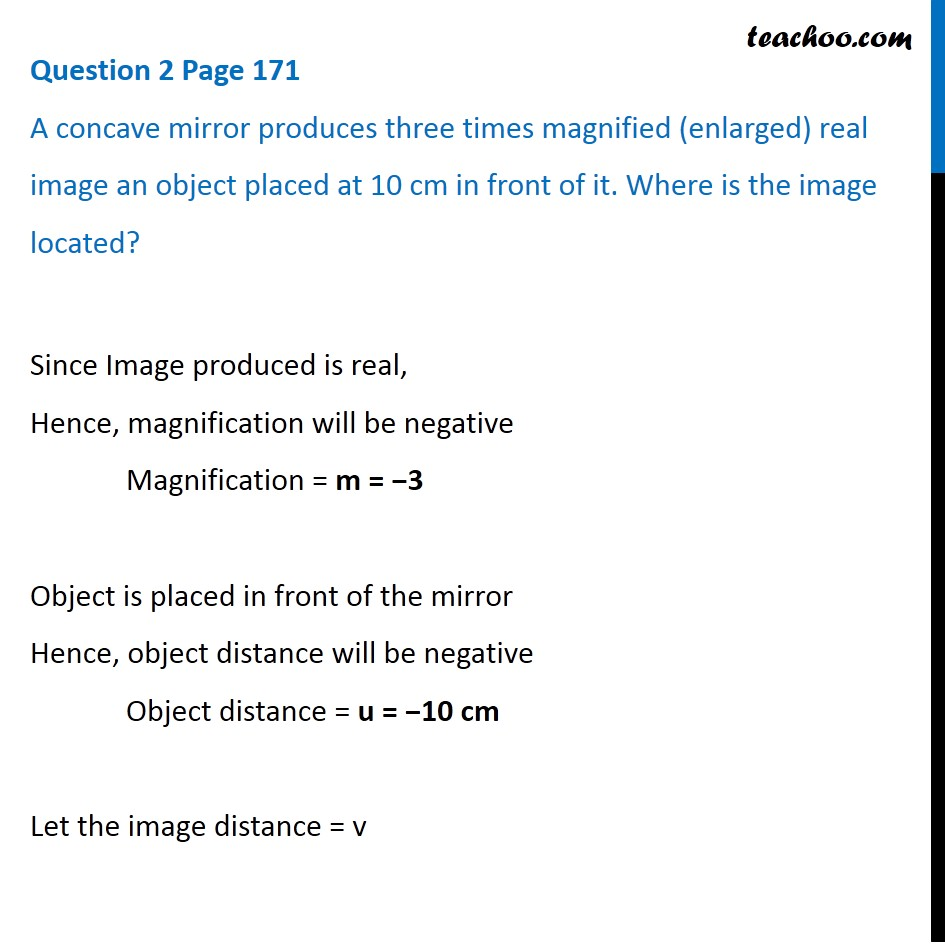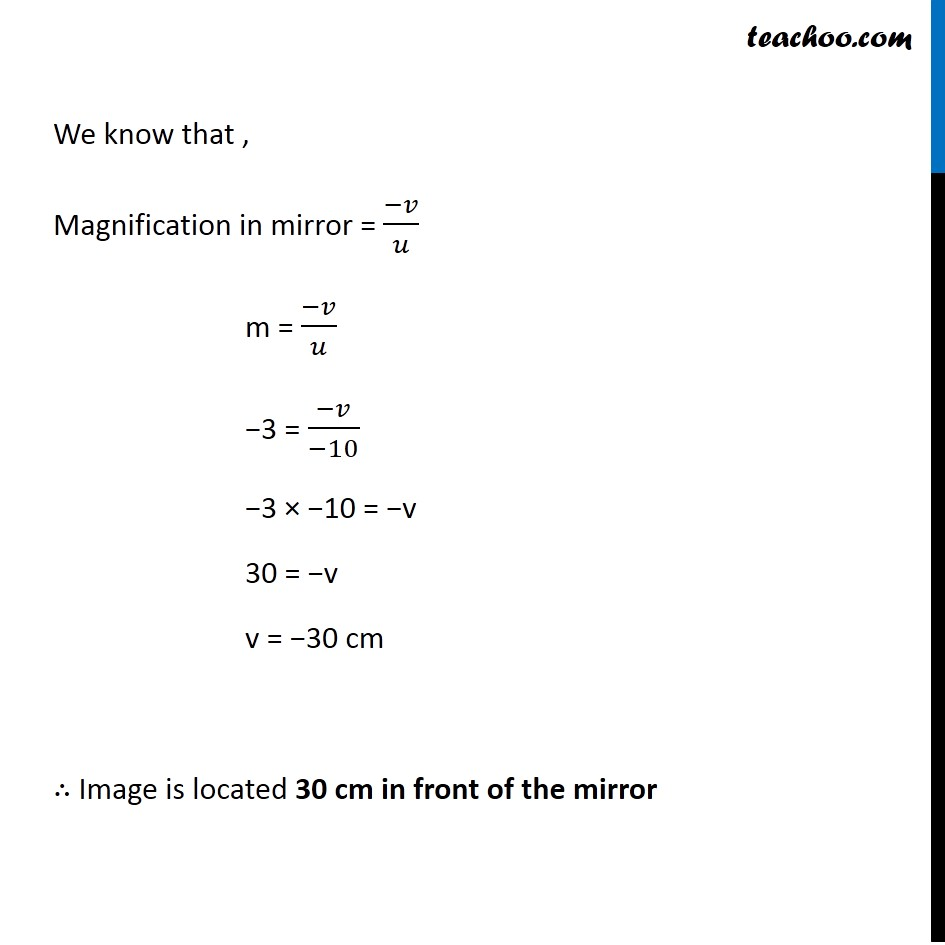Questions from Inside the chapter

Class 10
Chapter 10 Class 10 - Light - Reflection and Refraction

## A concave mirror produces three times magnified (enlarged) real image an object placed at 10 cm in front of it. Where is the image located?Learn in your speed, with individual attention - Teachoo Maths 1-on-1 Class

### Transcript

Question 2 Page 171 A concave mirror produces three times magnified (enlarged) real image an object placed at 10 cm in front of it. Where is the image located? Since Image produced is real, Hence, magnification will be negative Magnification = m = −3 Object is placed in front of the mirror Hence, object distance will be negative Object distance = u = −10 cm Let the image distance = v We know that , Magnification in mirror = (−𝑣)/𝑢 m = (−𝑣)/𝑢 −3 = (−𝑣)/(−10) −3 × −10 = −v 30 = −v v = −30 cm ∴ Image is located 30 cm in front of the mirror